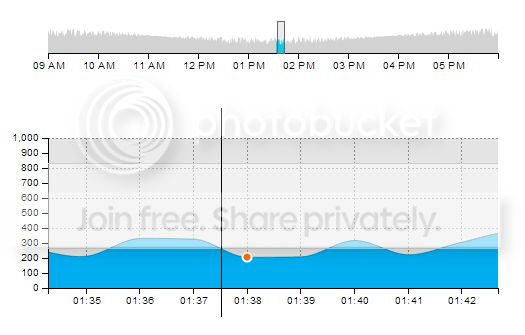# D3 Line-Path Intersection

I'm working with D3 and I've run into a bit of a problem. Basically, I have a small area chart whose domain changes based on a brush (similar to this example: http://bl.ocks.org/mbostock/1667367). I've created a line which can be dragged around and what I had hoped to do is provide a tooltip where this line intersected the area chart. I've created a function which bisects the data from the chart based on the x-value of the line and returns the closest data point... the issue is that this doesn't always line up with the reference line, especially if the brush has been set really small so there are only a few points being interpolated in the area chart. Here's an image of what I'm talking about.So, my question is it possible to somehow create a path-line intersection function which will determine the exact location where the two paths meet and return a value I can use for a tooltip? The function I'm currently using is below... but like I said, this doesn't seem to work well when the brush is set to a small area.

``````function ref_move(d) {
var x0 = x.invert(d.x -35),
i = bisectDate(data, x0, 1),
d0 = data[i - 1],
d1 = data[i],
d = x0 - d0.date > d1.date - x0 ? d1 : d0;

ref_tooltip.attr("transform", "translate(" + (x(d.date) + margin.left) + "," +   (y(d.Light) + light_offset) + ")");
}
``````

I've done some research on this issue and haven't come up with much of a solution. There is a library written by Kevin Lindsey which does some sort of intersection testing (http://www.kevlindev.com/geometry/2D/intersections/index.htm)... but it was written around 9 years ago... and not sure if it's still current. Additionally, there is an example where you can find a point along a path (http://bl.ocks.org/mbostock/1705868) but, I'm wondering how I can find the point along the path which is closes to the reference line? Does anyone have any ideas on how to solve this problem? Thanks in advance.

Thanks for the link. Interesting to get other ways to solve it. It turns out that I figured it out... essentially using some interpolation. You see I knew d0 and d1 which were the points on either side of the reference line that corresponded between data that I knew from my CSV file. So, basically I just needed to find out the position of the reference line between these two points to figure out a weighted percentage... and then multiply the two weights times the y value of the two known points and then you have it. My new function looks like this:

``````function ref_move(d) {
var x0 = x.invert(d.x -35),
i = bisectDate(data, x0, 1),
d0 = data[i - 1],
d1 = data[i],
span = (x(d1.date) - x(d0.date)),
weight = (x(x0) - x(d0.date))/span,
newy = (d1.Light * weight) + (d0.Light * (1.0-weight));

ref_tooltip.attr("transform", "translate(" + (x(x0) + margin.left) + "," + (y(newy) + light_offset) + ")");
}
``````

This seems to have fixed my issue. Thanks for the help. I'd be curious if there are other ways to solve it. Thanks again.

for the KenlinDel library I managed to to find the intersection points of two paths with using D3, but has issue with the other shapes, The actual solution I am doing is

``````chordshapes = Array()
svg.selectAll("g.Chords")
.data(data)
..attr("d", function (d,i) {
var shape = new Path() // from D2.js
//get the path
var pathStr = chrod(innerRadius, d.source, d.target)
//Initialize a new path object
shape.parseData(pathStr)
chordshapes[i] = shape;
return pathStr;
})
``````

So here the chordShapes containes the Path objects for each path, then when I have a click on for example a brush, I can create another shape for the brush rectangle and check the intersection points as follow

``````........
var intersect Intersection.intersectShapes(chordshapes[i], brushShape)
if(intersect.points.length >0) {
..........
}
``````